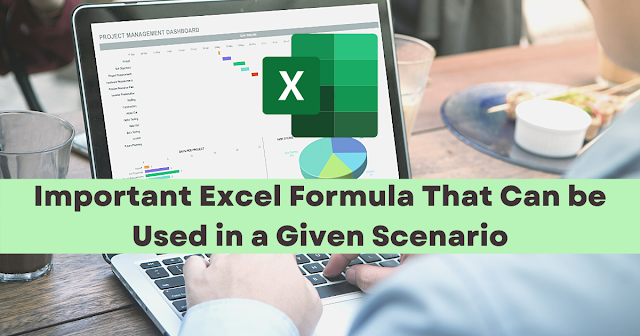# Important Excel Formula That Can be Used in a Given ScenarioExcel Spreadsheets speed up your work to be done, It helps you to be more time-efficient and effective in doing your tasks. Here are some tips that can help you out if you encounter these similar problems.

## Age Calculation

In calculating the age of the person, there are 2 formulas/methods that can be used. See the illustration below:
In method 1, it uses DATEDIF() function. The said syntax =DATEDIF(start date,end date,unit of time).
• Start Date- The starting date in a date format which you would like to calculate
• End Date- The ending date in a date format which would you like to calculate
• Unit- The unit of measurement. It can be in years("y"), months("m"), and days ("d")

In method 2, it uses TODAY() (See the illustration above)It determines the current date. It can be used as a value in calculating age. The 45 represents the years of age, while, .44 represents the months in a decimal form

## AutoSum Shortcut Key

Another way in using the Auto Sum Button which can be found in the toolbar, pressing "Alt" and "=" can also be used as an AutoSum.

## Use of Brackets

Sometimes you will need to use brackets, (also known as 'braces'), in the formula. This is to ensure that the calculations are performed in the order that you need. The need for brackets occurs when you mix + and = with divide and multiply.
Mathematically speaking, using the MDAS, multiplication and division operations will be calculated before Addition and Subtraction. In order to emphasize the Addition and Subtraction, use brackets and/braces.

## Instant Charts

You can create a chart quickly without having to use the chart button on the toolbar by pressing the function key "F11" while it is inside the range data.

## Formula Revealing

There is a shortcut in revealing the formula behind the selected cell/s. Just press Ctrl and ` . The ` symbol can be found on the left side of number 1

1.2.3.